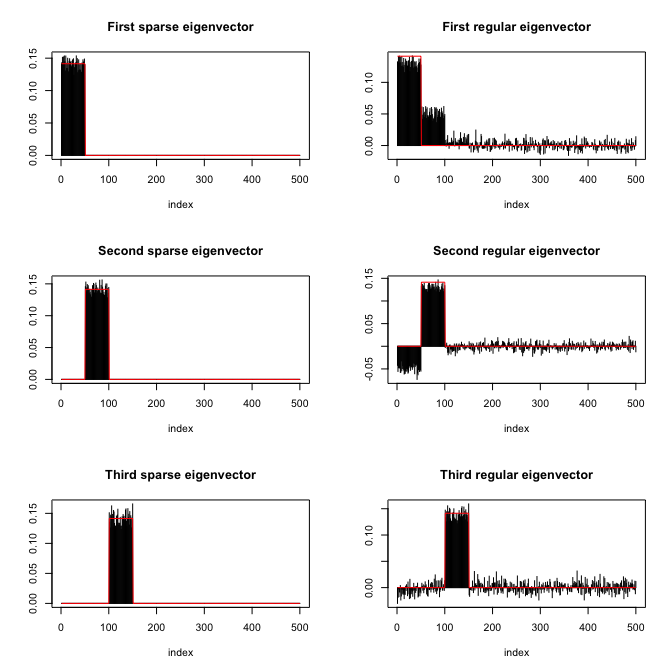This package provides functions to compute sparse eigenvectors (while keeping their orthogonality) or sparse PCA from either the covariance matrix or directly the data matrix.

## Installation

``````# Installation from GitHub
# install.packages("devtools")
devtools::install_github("dppalomar/sparseEigen")

# Get help
library(sparseEigen)
help(package="sparseEigen")
?spEigen``````

## Usage of `spEigen()`

We start by loading the package and generating synthetic data with sparse eigenvectors:

``````library(sparseEigen)
set.seed(42)

# parameters
m <- 500  # dimension
n <- 100  # number of samples
q <- 3  # number of sparse eigenvectors to be estimated
sp_card <- 0.1*m  # cardinality of each sparse eigenvector

# generate non-overlapping sparse eigenvectors
V <- matrix(0, m, q)
V[cbind(seq(1, q*sp_card), rep(1:q, each = sp_card))] <- 1/sqrt(sp_card)
V <- cbind(V, matrix(rnorm(m*(m-q)), m, m-q))
# keep first q eigenvectors the same (already orthogonal) and orthogonalize the rest
V <- qr.Q(qr(V))

# generate eigenvalues
lmd <- c(100*seq(from = q, to = 1), rep(1, m-q))

# generate covariance matrix from sparse eigenvectors and eigenvalues
R <- V %*% diag(lmd) %*% t(V)

# generate data matrix from a zero-mean multivariate Gaussian distribution
# with the constructed covariance matrix
X <- MASS::mvrnorm(n, rep(0, m), R)  # random data with underlying sparse structure``````

Then, we estimate the covariance matrix with `cov(X)` and compute its sparse eigenvectors:

``````# computation of sparse eigenvectors
res_standard <- eigen(cov(X))
res_sparse <- spEigen(cov(X), q)``````

We can assess how good the estimated eigenvectors are by computing the inner product with the original eigenvectors (the closer to 1 the better):

``````# show inner product between estimated eigenvectors and originals
abs(diag(t(res_standard\$vectors) %*% V[, 1:q]))  #for standard estimated eigenvectors
#>  0.9215392 0.9194898 0.9740871
abs(diag(t(res_sparse\$vectors) %*% V[, 1:q]))    #for sparse estimated eigenvectors
#>  0.9986937 0.9988146 0.9972078``````

Finally, the following plot shows the sparsity pattern of the eigenvectors (sparse computation vs. classical computation):## Usage of `spEigenCov()`

The function `spEigenCov()` requires more samples than the dimension (otherwise some regularization is required). Therefore, we generate data as previously with the only difference that we set the number of samples to be `n=600`.

Then, we compute the covariance matrix through the joint estimation of sparse eigenvectors and eigenvalues:

``````# computation of covariance matrix
res_sparse2 <- spEigenCov(cov(X), q)``````

Again, we can assess how good the estimated eigenvectors are by computing the inner product with the original eigenvectors:

``````# show inner product between estimated eigenvectors and originals
abs(diag(t(res_sparse2\$vectors[, 1:q]) %*% V[, 1:q]))    #for sparse estimated eigenvectors
#>  0.9997197 0.9996029 0.9992848``````

Finally, we can compute the error of the estimated covariance matrix (sparse eigenvector computation vs. classical computation):

``````# show error between estimated and true covariance
norm(cov(X) - R, type = 'F') #for sample covariance matrix
#>  48.42514
norm(res_sparse2\$cov - R, type = 'F') #for covariance with sparse eigenvectors
#>  25.74865``````